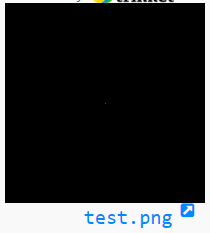﻿ NumPy: Convert a numpy array to an image - w3resource# NumPy: Convert a numpy array to an image

## NumPy: Array Object Exercise-109 with Solution

Write a NumPy program to convert a numpy array to an image. Display the image.

Sample Solution:

Python Code:

``````from PIL import Image
import numpy as np
img_w, img_h = 200, 200
data = np.zeros((img_h, img_w, 3), dtype=np.uint8)
data[100, 100] = [255, 0, 0]
img = Image.fromarray(data, 'RGB')
img.save('test.png')
img.show()
```
```

Sample Output:

``````

Python Code Editor:

Have another way to solve this solution? Contribute your code (and comments) through Disqus.

What is the difficulty level of this exercise?

Test your Python skills with w3resource's quiz

﻿

## Python: Tips of the Day

List comprehension:

```>>> m = [x ** 2 for x in range(5)]
>>> m
[0, 1, 4, 9, 16]
```